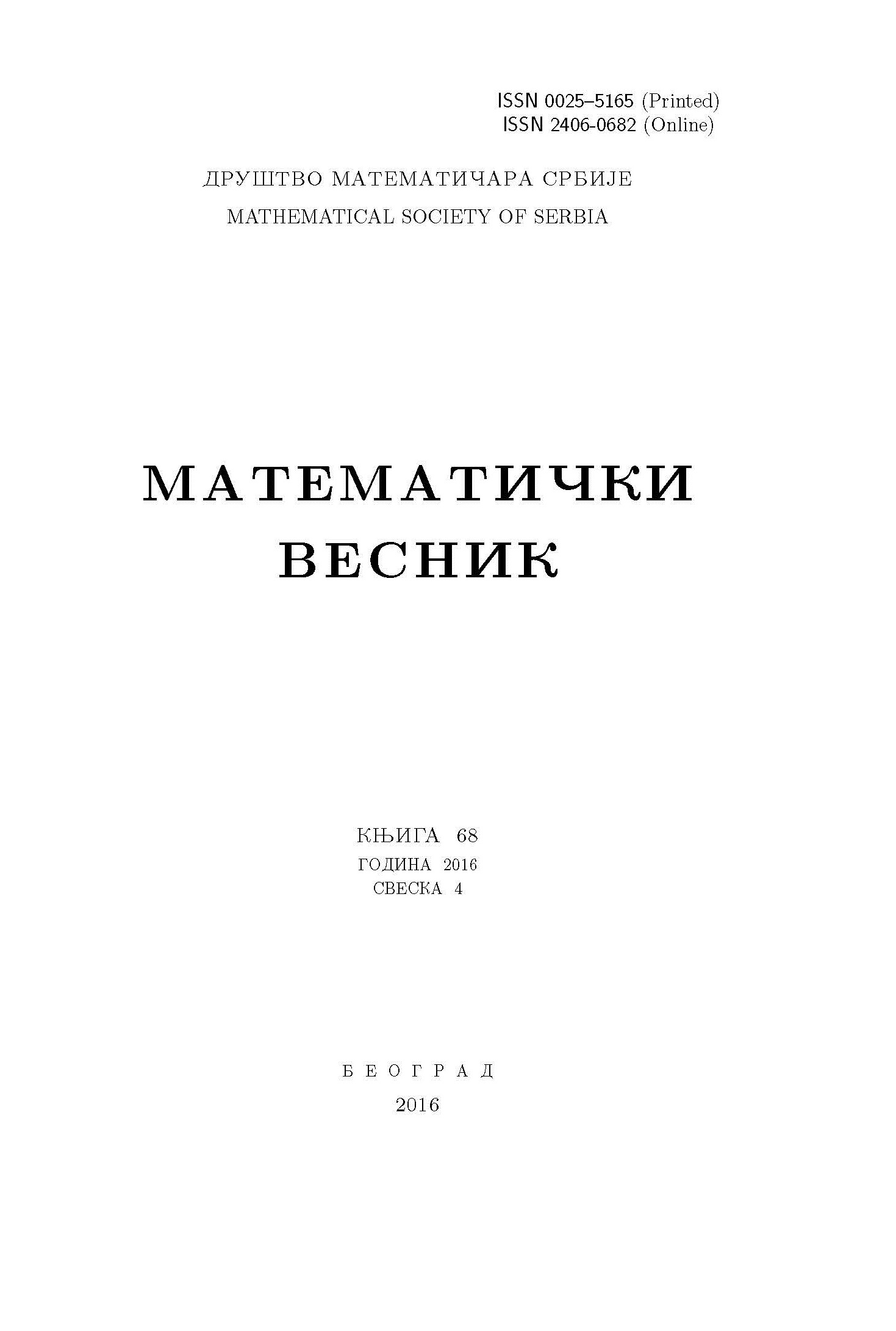﻿ Matematički Vesnik ﻿
 MATEMATIČKI VESNIK МАТЕМАТИЧКИ ВЕСНИКHARNACK ESTIMATES FOR THE POROUS MEDIUM EQUATION WITH POTENTIAL UNDER GEOMETRIC FLOW S. Azami AbstractLet $(M, g(t))$, $t\in[0,T)$ be a closed Riemannian $n$-manifold whose Riemannian metric $g(t)$ evolves by the geometric flow $\frac{\partial }{\partial t} g_{ij}=-2S_{ij}$, where $S_{ij}(t)$ is a symmetric two-tensor on $(M,g(t))$. We discuss differential Harnack estimates for positive solution to the porous medium equation with potential, $\frac{\partial u}{\partial t}=\Delta u^{p}+S u$, where $S=g^{ij}S_{ij}$ is the trace of $S_{ij}$, on time-dependent Riemannian metric evolving by the above geometric flow.Keywords: Harnack estimates; geometric flow; porous medium equation. MSC: 53C21, 53C44, 58J35 Pages:  15$-$25 Volume  74 ,  Issue  1 ,  2022

﻿